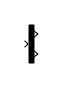# Demux

Extract and output elements of virtual vector signal

•Libraries:
HDL Coder / Commonly Used Blocks
HDL Coder / Signal Routing

## Description

The Demux block extracts the components of an input vector signal and outputs separate signals. The output signal ports are ordered from top to bottom.

## Examples

expand all

You can use the Demux block to distribute an input signal evenly over the desired number of outputs. For an input vector of length 6, when you set the Number of outputs parameter to `3`, the Demux block creates three output signals, each of size 2.When using the Demux block to extract and output elements from a vector input, you can use -1 in a vector expression to indicate that the block dynamically sizes the corresponding port. When a vector expression comprises both positive values and -1 values, the block assigns as many elements as needed to the ports with positive values. The block distributes the remaining elements as evenly as possible over the ports with -1 values.

In this example, the Number of outputs parameter of the Demux block is set to [-1, 3, -1]. Thus, the block outputs three signals where the second signal always has three elements. The sizes of the first and third signals depend on the size of the input signal. For an input vector with seven elements, the Demux block outputs two elements on the first port, three elements on the second port, and two elements on the third port.## Ports

### Input

expand all

Vector input signal from which the Demux block selects scalar signals or smaller vectors.

Data Types: `single` | `double` | `half` | `int8` | `int16` | `int32` | `int64` | `uint8` | `uint16` | `uint32` | `uint64` | `Boolean` | `fixed point` | `enumerated` | `image`

### Output

expand all

Output signals extracted from the input vector. The output signal ports are ordered from top to bottom. For a description of the port order for various block orientations, see Identify Port Location on Rotated or Flipped Block.

Data Types: `single` | `double` | `half` | `int8` | `int16` | `int32` | `int64` | `uint8` | `uint16` | `uint32` | `uint64` | `Boolean` | `fixed point` | `enumerated` | `image`

## Parameters

expand all

Specify the number of outputs and, optionally, the dimensionality of each output port.

The value can be a scalar specifying the number of outputs or a vector whose elements specify the widths of the block output ports. The block determines the size of its outputs from the size of the input signal and the value of the Number of outputs parameter.

If you specify a scalar for the Number of outputs parameter, and all of the output ports are connected, as you draw a new signal line close to the output side of a Demux block, the software adds a port and updates the Number of outputs parameter.

For an input vector of width `n`, this table describes what the block outputs.

`p = n`

`p` scalar signals

If the input is a three-element vector and you specify three outputs, the block outputs three scalar signals.

`p > n`

Error

This value is not supported.

`p < n`

```n mod p = 0```

`p` vector signals each having `n/p` elements

If the input is a six-element vector and you specify three outputs, the block outputs three two-element vectors.

`p < n`

```n mod p = m```

`m` vector signals each having `(n/p)+1` elements and `p-m` signals having `n/p `elements

If the input is a five-element vector and you specify three outputs, the block outputs two two-element vector signals and one scalar signal.

`[p`1``` p```2``` ... p```m`]`

`p`1`+p`2`+...+p`m`=n`

`p`i``` > 0```

`m` vector signals having widths `p1`, `p2`, ... `pm`

If the input is a five-element vector and you specify ```[3, 2]``` as the output, the block outputs three of the input elements on one port and the other two elements on the other port.

An array that has one or more of `m` elements with a value of `-1`, which specifies that the software infers the size for the element.

For example, suppose that you have a four-element array with a total width of 14 and you specify the parameter to be `[p`1``` p```2` -1```` p```4`]`.

The value for the third element (the `-1` element) is ```14 - ``````(p1 + p2 + p4)```

`m` vector signals

If `p`i is greater than zero, the corresponding output has width `p`i. If `p`i is -1, the width of the corresponding output is computed dynamically.

`[p`1``` p```2``` ... p```m`]`

`p`1`+p`2`+...+p`m`!=n`

`p`i``` = > 0```

Error

This value is not supported.

If you specify a number of outputs that is smaller than the number of input elements, the block distributes the elements as evenly as possible over the outputs.

#### Programmatic Use

To set the block parameter value programmatically, use the `set_param` function.

 Parameter: `Outputs` Values: `'2'` (default) | character vector | string scalar Data Types: `char` | `string`

Example: `set_param(gcb,'Outputs','4')`

By default, the block icon is a solid bar of the block foreground color. To display the icon as a box containing the block type name, select `none`.

#### Programmatic Use

To set the block parameter value programmatically, use the `set_param` function.

 Parameter: `DisplayOption` Values: `'bar'` (default) | `'none'`

Example: `set_param(gcb,'DisplayOption','none')`

## Block Characteristics

 Data Types `Boolean` | `double` | `enumerated` | `fixed point` | `half` | `integer` | `single` Direct Feedthrough `yes` Multidimensional Signals `no` Variable-Size Signals `no` Zero-Crossing Detection `no`

## Version History

Introduced before R2006a